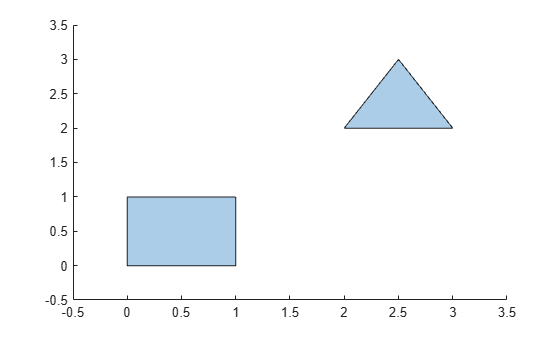# sortboundaries

Sort `polyshape` boundaries

## Syntax

``polyout = sortboundaries(polyin,criterion,direction)``
``polyout = sortboundaries(polyin,'centroid',direction,'ReferencePoint',point)``

## Description

example

````polyout = sortboundaries(polyin,criterion,direction)` returns a `polyshape` object whose boundaries are sorted according to the specified `criterion` and `direction`. For example, `polyout = sortboundaries(polyin,'area','ascend')` returns a `polyshape` whose boundaries are the same as `polyin`. `polyout` lists the boundaries in ascending order by their area.```
````polyout = sortboundaries(polyin,'centroid',direction,'ReferencePoint',point)` returns a `polyshape` whose boundaries are sorted based on the distance of the centroid of each boundary to a reference point.```

## Examples

collapse all

Sort the boundaries of a polygon according to their number of sides in order to access a single boundary at a time.

Create a polygon containing two boundaries, one with three sides and one with four sides.

```x1 = [0 1 1 0]; y1 = [0 0 1 1]; x2 = [2 3 2.5]; y2 = [2 2 3]; polyin = polyshape({x1,x2},{y1,y2}); plot(polyin)```Sort the boundaries in descending order according to their number of sides, so that the four-sided boundary is indexed first in the output `polyshape` object.

`polyout = sortboundaries(polyin,'numsides','descend');`

You now can reference the four-sided boundary based on its index, for example when you want to access the boundary's vertices or compute its area.

`[x,y] = boundary(polyout,1)`
```x = 5×1 0 0 1 1 0 ```
```y = 5×1 0 1 1 0 0 ```
`area(polyout,1)`
```ans = 1 ```

## Input Arguments

collapse all

Input `polyshape`, specified as a scalar, vector, matrix, or multidimensional array.

Data Types: `polyshape`

Sort criterion, specified as one of these values:

• `'area'` — Sort by boundary area.

• `'perimeter'` — Sort by boundary perimeter.

• `'numsides'` — Sort by the number of sides of each boundary.

• `'centroid'` — Sort by the distance from the centroid of each boundary to the reference point (0,0).

Sort direction, specified as `'ascend'` or `'descend'`.

Reference point, specified as a two-element row vector. The first element is the x-coordinate of the reference point, and the second element is the y-coordinate.